# SSAO

Note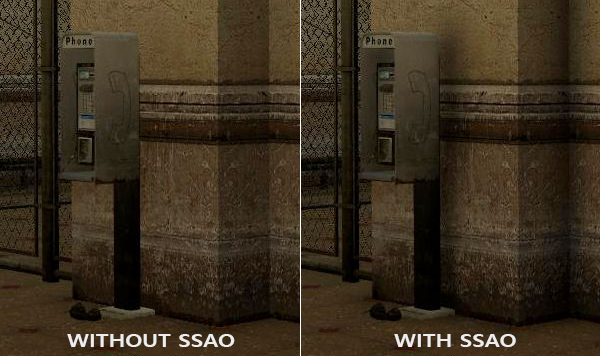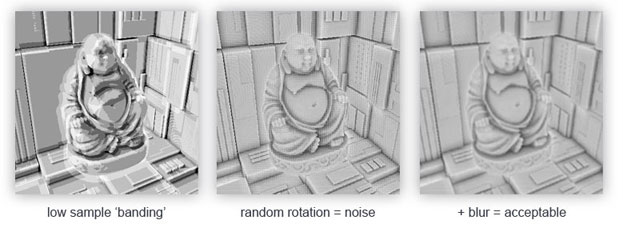Crytek公司开发的SSAO技术会产生一种特殊的视觉风格。因为使用的采样核心是一个球体，它导致平整的墙面也会显得灰蒙蒙的，因为核心中一半的样本都会在墙这个几何体上。下面这幅图展示了孤岛危机的SSAO，它清晰地展示了这种灰蒙蒙的感觉：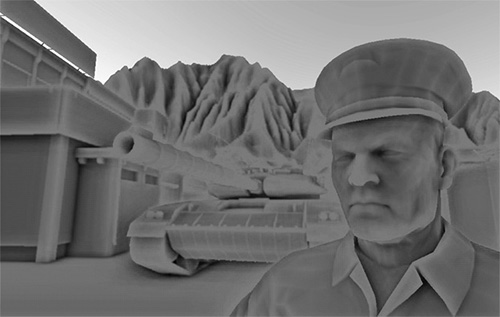## 样本缓冲

SSAO需要获取几何体的信息，因为我们需要一些方式来确定一个片段的遮蔽因子。对于每一个片段，我们将需要这些数据：

• 逐片段位置向量
• 逐片段的法线向量
• 逐片段的反射颜色
• 采样核心
• 用来旋转采样核心的随机旋转矢量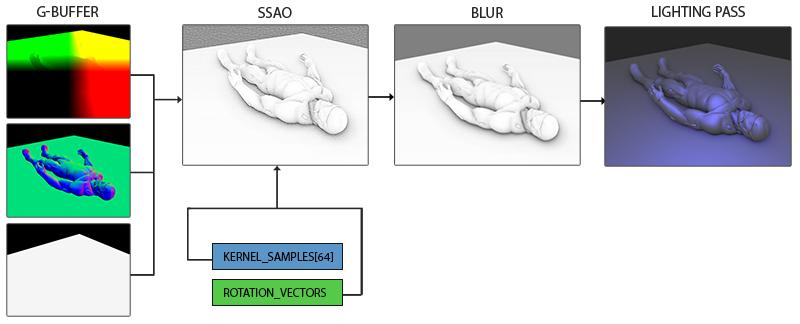Important

#version 330 core
layout (location = 0) out vec4 gPositionDepth;
layout (location = 1) out vec3 gNormal;
layout (location = 2) out vec4 gAlbedoSpec;

in vec2 TexCoords;
in vec3 FragPos;
in vec3 Normal;

const float NEAR = 0.1; // 投影矩阵的近平面
const float FAR = 50.0f; // 投影矩阵的远平面
float LinearizeDepth(float depth)
{
float z = depth * 2.0 - 1.0; // 回到NDC
return (2.0 * NEAR * FAR) / (FAR + NEAR - z * (FAR - NEAR));
}

void main()
{
// 储存片段的位置矢量到第一个G缓冲纹理
gPositionDepth.xyz = FragPos;
// 储存线性深度到gPositionDepth的alpha分量
gPositionDepth.a = LinearizeDepth(gl_FragCoord.z);
// 储存法线信息到G缓冲
gNormal = normalize(Normal);
// 和漫反射颜色
gAlbedoSpec.rgb = vec3(0.95);
}


Important

gPositionDepth颜色缓冲纹理被设置成了下面这样：

glGenTextures(1, &gPositionDepth);
glBindTexture(GL_TEXTURE_2D, gPositionDepth);
glTexImage2D(GL_TEXTURE_2D, 0, GL_RGBA16F, SCR_WIDTH, SCR_HEIGHT, 0, GL_RGBA, GL_FLOAT, NULL);
glTexParameteri(GL_TEXTURE_2D, GL_TEXTURE_MIN_FILTER, GL_NEAREST);
glTexParameteri(GL_TEXTURE_2D, GL_TEXTURE_MAG_FILTER, GL_NEAREST);
glTexParameteri(GL_TEXTURE_2D, GL_TEXTURE_WRAP_S, GL_CLAMP_TO_EDGE);
glTexParameteri(GL_TEXTURE_2D, GL_TEXTURE_WRAP_T, GL_CLAMP_TO_EDGE);


## 法向半球std::uniform_real_distribution<GLfloat> randomFloats(0.0, 1.0); // 随机浮点数，范围0.0 - 1.0
std::default_random_engine generator;
std::vector<glm::vec3> ssaoKernel;
for (GLuint i = 0; i < 64; ++i)
{
glm::vec3 sample(
randomFloats(generator) * 2.0 - 1.0,
randomFloats(generator) * 2.0 - 1.0,
randomFloats(generator)
);
sample = glm::normalize(sample);
sample *= randomFloats(generator);
GLfloat scale = GLfloat(i) / 64.0;
ssaoKernel.push_back(sample);
}


   ...[接上函数]
scale = lerp(0.1f, 1.0f, scale * scale);
sample *= scale;
ssaoKernel.push_back(sample);
}


lerp被定义为：

GLfloat lerp(GLfloat a, GLfloat b, GLfloat f)
{
return a + f * (b - a);
}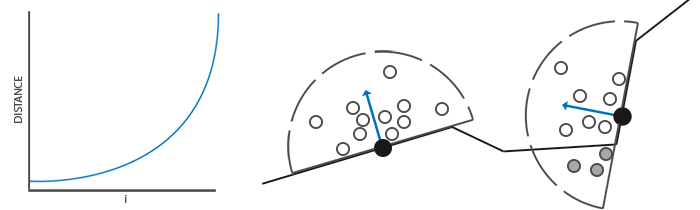## 随机核心转动

std::vector<glm::vec3> ssaoNoise;
for (GLuint i = 0; i < 16; i++)
{
glm::vec3 noise(
randomFloats(generator) * 2.0 - 1.0,
randomFloats(generator) * 2.0 - 1.0,
0.0f);
ssaoNoise.push_back(noise);
}


GLuint noiseTexture;
glGenTextures(1, &noiseTexture);
glBindTexture(GL_TEXTURE_2D, noiseTexture);
glTexImage2D(GL_TEXTURE_2D, 0, GL_RGB16F, 4, 4, 0, GL_RGB, GL_FLOAT, &ssaoNoise);
glTexParameteri(GL_TEXTURE_2D, GL_TEXTURE_MIN_FILTER, GL_NEAREST);
glTexParameteri(GL_TEXTURE_2D, GL_TEXTURE_MAG_FILTER, GL_NEAREST);
glTexParameteri(GL_TEXTURE_2D, GL_TEXTURE_WRAP_S, GL_REPEAT);
glTexParameteri(GL_TEXTURE_2D, GL_TEXTURE_WRAP_T, GL_REPEAT);


## SSAO着色器

SSAO着色器在2D的铺屏四边形上运行，它对于每一个生成的片段计算遮蔽值(为了在最终的光照着色器中使用)。由于我们需要存储SSAO阶段的结果，我们还需要在创建一个帧缓冲对象：

GLuint ssaoFBO;
glGenFramebuffers(1, &ssaoFBO);
glBindFramebuffer(GL_FRAMEBUFFER, ssaoFBO);
GLuint ssaoColorBuffer;

glGenTextures(1, &ssaoColorBuffer);
glBindTexture(GL_TEXTURE_2D, ssaoColorBuffer);
glTexImage2D(GL_TEXTURE_2D, 0, GL_RED, SCR_WIDTH, SCR_HEIGHT, 0, GL_RGB, GL_FLOAT, NULL);
glTexParameteri(GL_TEXTURE_2D, GL_TEXTURE_MIN_FILTER, GL_NEAREST);
glTexParameteri(GL_TEXTURE_2D, GL_TEXTURE_MAG_FILTER, GL_NEAREST);
glFramebufferTexture2D(GL_FRAMEBUFFER, GL_COLOR_ATTACHMENT0, GL_TEXTURE_2D, ssaoColorBuffer, 0);


// 几何处理阶段: 渲染到G缓冲中
glBindFramebuffer(GL_FRAMEBUFFER, gBuffer);
[...]
glBindFramebuffer(GL_FRAMEBUFFER, 0);

// 使用G缓冲渲染SSAO纹理
glBindFramebuffer(GL_FRAMEBUFFER, ssaoFBO);
glClear(GL_COLOR_BUFFER_BIT);
glActiveTexture(GL_TEXTURE0);
glBindTexture(GL_TEXTURE_2D, gPositionDepth);
glActiveTexture(GL_TEXTURE1);
glBindTexture(GL_TEXTURE_2D, gNormal);
glActiveTexture(GL_TEXTURE2);
glBindTexture(GL_TEXTURE_2D, noiseTexture);
glUniformMatrix4fv(projLocation, 1, GL_FALSE, glm::value_ptr(projection));
glBindFramebuffer(GL_FRAMEBUFFER, 0);

// 光照处理阶段: 渲染场景光照
glClear(GL_COLOR_BUFFER_BIT | GL_DEPTH_BUFFER_BIT);
[...]
glActiveTexture(GL_TEXTURE3);
glBindTexture(GL_TEXTURE_2D, ssaoColorBuffer);
[...]


shaderSSAO这个着色器将对应G缓冲纹理(包括线性深度)，噪声纹理和法向半球核心样本作为输入参数：

#version 330 core
out float FragColor;
in vec2 TexCoords;

uniform sampler2D gPositionDepth;
uniform sampler2D gNormal;
uniform sampler2D texNoise;

uniform vec3 samples;
uniform mat4 projection;

// 屏幕的平铺噪声纹理会根据屏幕分辨率除以噪声大小的值来决定
const vec2 noiseScale = vec2(800.0/4.0, 600.0/4.0); // 屏幕 = 800x600

void main()
{
[...]
}


vec3 fragPos = texture(gPositionDepth, TexCoords).xyz;
vec3 normal = texture(gNormal, TexCoords).rgb;
vec3 randomVec = texture(texNoise, TexCoords * noiseScale).xyz;


vec3 tangent = normalize(randomVec - normal * dot(randomVec, normal));
vec3 bitangent = cross(normal, tangent);
mat3 TBN = mat3(tangent, bitangent, normal);


float occlusion = 0.0;
for(int i = 0; i < kernelSize; ++i)
{
// 获取样本位置
vec3 sample = TBN * samples[i]; // 切线->观察空间
sample = fragPos + sample * radius;

[...]
}


vec4 offset = vec4(sample, 1.0);
offset = projection * offset; // 观察->裁剪空间
offset.xyz /= offset.w; // 透视划分
offset.xyz = offset.xyz * 0.5 + 0.5; // 变换到0.0 - 1.0的值域


float sampleDepth = -texture(gPositionDepth, offset.xy).w;


occlusion += (sampleDepth >= sample.z ? 1.0 : 0.0);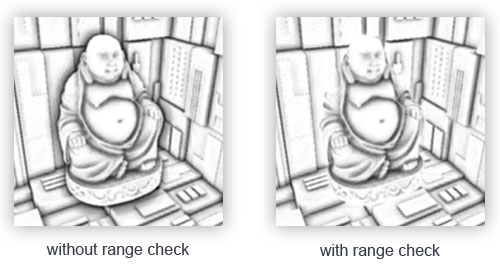float rangeCheck = smoothstep(0.0, 1.0, radius / abs(fragPos.z - sampleDepth));
occlusion += (sampleDepth >= sample.z ? 1.0 : 0.0) * rangeCheck;}
occlusion = 1.0 - (occlusion / kernelSize);
FragColor = occlusion;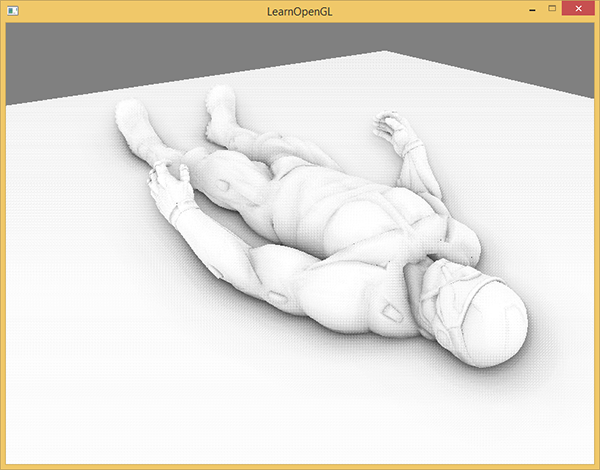## 环境遮蔽模糊

GLuint ssaoBlurFBO, ssaoColorBufferBlur;
glGenFramebuffers(1, &ssaoBlurFBO);
glBindFramebuffer(GL_FRAMEBUFFER, ssaoBlurFBO);
glGenTextures(1, &ssaoColorBufferBlur);
glBindTexture(GL_TEXTURE_2D, ssaoColorBufferBlur);
glTexImage2D(GL_TEXTURE_2D, 0, GL_RED, SCR_WIDTH, SCR_HEIGHT, 0, GL_RGB, GL_FLOAT, NULL);
glTexParameteri(GL_TEXTURE_2D, GL_TEXTURE_MIN_FILTER, GL_NEAREST);
glTexParameteri(GL_TEXTURE_2D, GL_TEXTURE_MAG_FILTER, GL_NEAREST);
glFramebufferTexture2D(GL_FRAMEBUFFER, GL_COLOR_ATTACHMENT0, GL_TEXTURE_2D, ssaoColorBufferBlur, 0);


#version 330 core
in vec2 TexCoords;
out float fragColor;

uniform sampler2D ssaoInput;

void main() {
vec2 texelSize = 1.0 / vec2(textureSize(ssaoInput, 0));
float result = 0.0;
for (int x = -2; x < 2; ++x)
{
for (int y = -2; y < 2; ++y)
{
vec2 offset = vec2(float(x), float(y)) * texelSize;
result += texture(ssaoInput, TexCoords + offset).r;
}
}
fragColor = result / (4.0 * 4.0);
}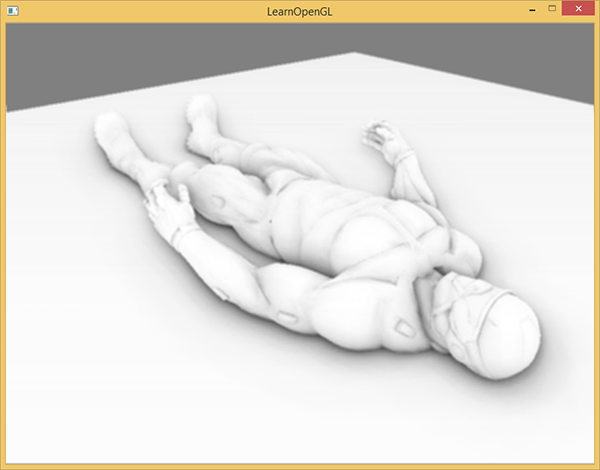## 应用环境遮蔽

#version 330 core
out vec4 FragColor;
in vec2 TexCoords;

uniform sampler2D gPositionDepth;
uniform sampler2D gNormal;
uniform sampler2D gAlbedo;
uniform sampler2D ssao;

struct Light {
vec3 Position;
vec3 Color;

float Linear;
};
uniform Light light;

void main()
{
// 从G缓冲中提取数据
vec3 FragPos = texture(gPositionDepth, TexCoords).rgb;
vec3 Normal = texture(gNormal, TexCoords).rgb;
vec3 Diffuse = texture(gAlbedo, TexCoords).rgb;
float AmbientOcclusion = texture(ssao, TexCoords).r;

// Blinn-Phong (观察空间中)
vec3 ambient = vec3(0.3 * AmbientOcclusion); // 这里我们加上遮蔽因子
vec3 lighting  = ambient;
vec3 viewDir  = normalize(-FragPos); // Viewpos 为 (0.0.0)，在观察空间中
// 漫反射
vec3 lightDir = normalize(light.Position - FragPos);
vec3 diffuse = max(dot(Normal, lightDir), 0.0) * Diffuse * light.Color;
// 镜面
vec3 halfwayDir = normalize(lightDir + viewDir);
float spec = pow(max(dot(Normal, halfwayDir), 0.0), 8.0);
vec3 specular = light.Color * spec;
// 衰减
float dist = length(light.Position - FragPos);
float attenuation = 1.0 / (1.0 + light.Linear * dist + light.Quadratic * dist * dist);
diffuse  *= attenuation;
specular *= attenuation;
lighting += diffuse + specular;

FragColor = vec4(lighting, 1.0);
}


(除了将其改到观察空间)对比于之前的光照实现，唯一的真正改动就是场景环境分量与AmbientOcclusion值的乘法。通过在场景中加入一个淡蓝色的点光源，我们将会得到下面这个结果：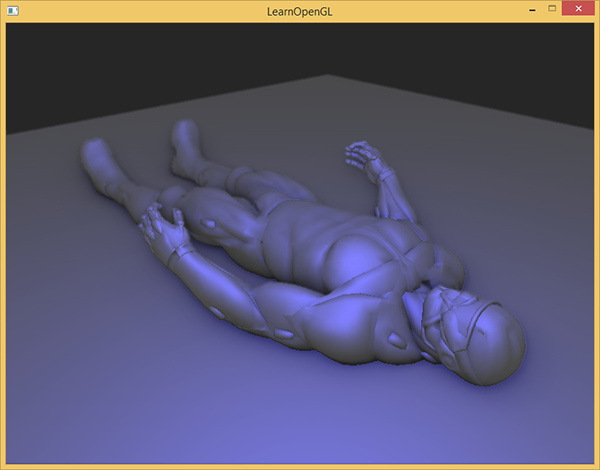occlusion = 1.0 - (occlusion / kernelSize);
FragColor = pow(occlusion, power);


## 附加资源

• SSAO教程：John Chapman优秀的SSAO教程；本教程很大一部分代码和技巧都是基于他的文章
• 了解你的SSAO效果：关于提高SSAO特定效果的一篇很棒的文章
• 深度值重构SSAO：OGLDev的一篇在SSAO之上的拓展教程，它讨论了通过仅仅深度值重构位置矢量，节省了存储开销巨大的位置矢量到G缓冲的过程